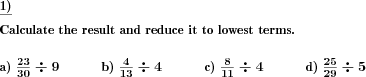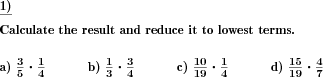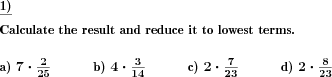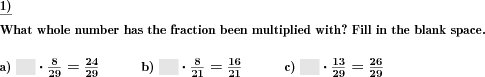Custom math worksheets at your fingertips# Details for problem "Decimal fraction divide by whole number"

Quickname: 1454

Elementary School, Primary School, Junior High School, Middle School, High School.

## Summary

A decimal fraction has to be divided by a whole number.

## Example## Description

A decimal fraction is one of the basic topics to learn in Maths and this worksheet provides practice problems related to decimal fractions divided by a whole number. A decimal fraction is presented, it is the dividend in a division problem. A divisor is a whole number. Practicing these problems is necessary for learners to grasp the division with decimal fractions skills. Assign these worksheets to struggle students for independent practice/ homework and let them grasp the skills. These worksheets can also be used in variation for assessment purposes. Along with this main objective, grade 6 to grade 8 learners will also achieve the following set of targets.

1. Revise the concepts of division.
2. Reinforce the concept of division by a whole number.
3. Use mental maths times table skills.
4. Master the division of a decimal fraction by a whole number.
5. Apply this skill to real-world problems.

Personalize these worksheets and serve to differentiated ability learners.

• The number of places before the decimal point can be selected.
• The number of decimals can be selected for the dividend.
• For the divisor, the number range can be specified.
• The number of problems can be selected.

The answer key consists of all problems with solutions.

Download free printable worksheets for this math problem here. The worksheet contains the problems only, the solution sheet includes the answers. Just click on the respective link.

•Worksheet 1Solution sheet with answers
•Worksheet 2Solution sheet with answers
•Worksheet 3Solution sheet with answers

If you can not see the solution sheets for download, they may be filtered out by an ad blocker that you may have installed. If this is the case, please allow ads for this page and reload the page. The solution sheets will then reappear.

• Do these sample worksheets do not really fit?
• Do you need more math worksheets, with a different level of difficulty?
• Would you like to combine different problems on a worksheet and adjust them to your needs?
• As a teacher, you can put together your own worksheets using the automatically generated math problems provided.
With a free initial credit, you can start creating your own math worksheets in a few minutes.

You can try it for free! Register here, to create custom worksheets now!

## Customization options for this problem

Parameter
Possible values
Number of problems
1, 2, 3, 4, 5, 6, 7, 8, 9, 10
Places
0, 1, 2, 3
Decimal places
1, 2, 3, 4
Decimals 0 up to pos
0, 1, 2, 3
Number range whole no
10, 20, 30, 40, 50, 100, 200, 500, 1000, 10000, 100000

## Similar problems

Remark
Description
Special case divisor is power of ten
A decimal fraction has to be divided by a power of ten.
Divisor is a fraction, too
A decimal fraction has to be divided by another decimal fraction.

## Other types of problems that appear on worksheets with this problem:

Relevance
Name
Description
Quickname
Example
****
Divide a fraction by a whole number
A fraction has to be divided by a whole number.****
Multiply fractions
Multiply two fractions.****
Multiply fractions by whole numbers
A fraction has to multiplied by a whole number.****
Fractions multiplication by whole numbers fill blanks
What whole number has be fraction been multiplied with?